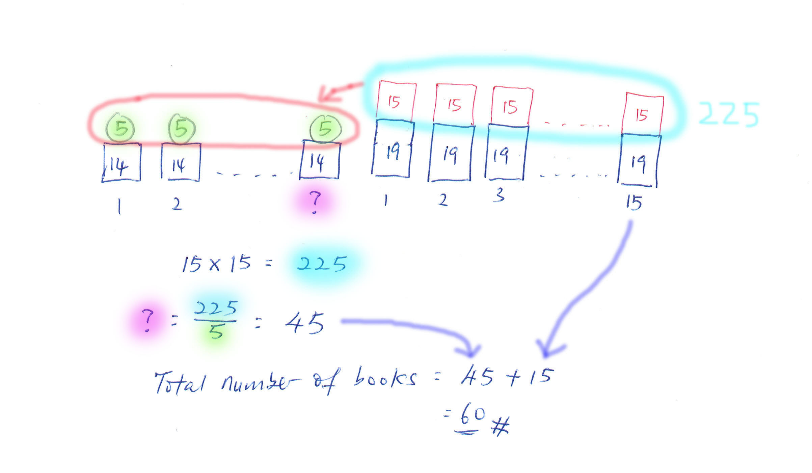# Question

Danson sold some books at an average cost of \$14. When he sold 15 more books at an average cost of \$34, the average cost of all the books sold became \$19. How many books did Danson sell in all?

Source: Greenridge PrimaryIf you prefer some pictures …

0 Replies 0 Likes

Using weightage.

The \$14 books is \$5 away from the new average at \$19. And the \$34 is \$15 away from the new average.

Since the \$14 books is nearer to \$19, therefore it has more weights, i.e more number of books.

How is the proportion of books spread between \$14 and \$34 books? It is by the gaps, \$5 and \$15.

Ratio of \$14 books : \$34 books  => 15 : 5  , \$14 books has more weights, therefore they have more proportion.

If \$34 books are 15 books, base on the above ratio (15 : 5) ,  there are 45 books of \$14.

Altogether is 45 +15 = 60 books ##

Hi Ender, I think this weightage is higher level concept not taught at P6… But good for kids strong in Maths to have a play with numbers.

Maybe present this as equating of “losses” and “gains” for easier understanding?

Gain by \$14-Ave cost books (to \$19) =  loss by \$34-Ave cost books (to \$19)

\$5 X ?   = \$15 X 15 books

\$5 X 45 cheaper books = \$15 X 15 more expensive books

Total= 60 books#

I agree this concept may be higher level, but since this is a Math Olympiad question, guess it will benefit those who are already a math olympian with the concept that the more samples you have for a value, the more it will influence the average towards them and the influence is inversely proportioned by the gaps between the average.

For this question the basic formula is 14(n) + 34(n2) /(n1 + n2). A student who understand this concept will understand that n and n1 are the weigths, or influencer. And the ratio is actually derived from this basic formula they learn in school.

14(n) + 34(n2) /(n1 + n2) = 19
14(n) + 34 (n2) = 19n1 + 19n2
34(n2) – 19 (n2) = 19(n) – 14(n) , take note, that’s the gap, (34 – 19) and (19 – 14)
15n2 = 5n
n/n2 = 15/5 , proof of ratio are the inversely proportional to the gaps .

The advantage is a faster solution. I see a two liner working for a math olympian.
The only useful info is the gaps , 15 : 5 in favor of the closer one to the average
45 : 15 , total =60 ###

Oic. Thanks for pointing out. 🙂

Yah I overlooked that it’s Maths Olympiad question, which I’m not familiar with or the concepts to know and apply. And yes, agree it’s Super fast to do for speed is needed at MO.

0 Replies 0 Likes

Let the number of books sold at first be “u”

Total cost of the 15 books –>34 x 15 = 510

Price of books sold at first–>14 x u = 14u

510 + 14u = (u + 15) x 19

510 + 14u = 19u + 285

19u – 14u = 510 – 285

5u = 225

1u = 45

Total number of books –>45 + 15 = 60

0 Replies 0 Likes

An alternative.

number of books x 14 + (15 x 34)/(number of books + 15) = 19
14 x number of books + 510 = 19 x number of books + 285
number of books = (510 – 285)/(19 – 14) = 45
45 + 15 = 60

Ans : 60 books.

0 Replies 0 Likes

Think about changes. Danwon can sell me:

Batch 1 of books at \$14each, unknown no. of books and

Batch 2 of books at \$34 each, 15 books.

Or one time sell whole lot of books at \$19 each, total unknown no. of books

Let’s view batch 2 as this: 15 books at \$14 each AND excess \$20×15 to redistribute to total no. of books to raise average costs from \$14 to \$19 (increase by \$5 each)

\$34-\$14 =\$20

(20 X 15) /5 = 60 books#

0 Replies 1 Like